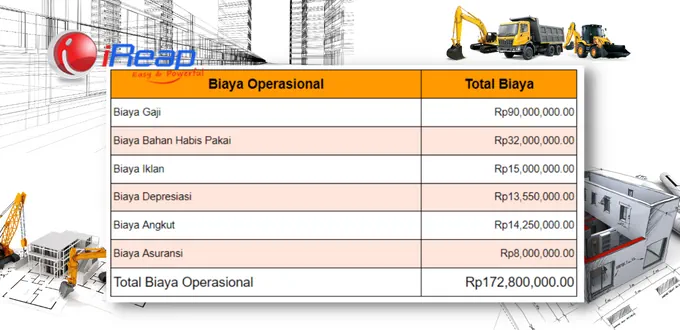# How to Calculate Operational Costs?The way to calculate operating costs is to add production costs to all operating expenses. For more details, let’s see how to calculate operational costs along with the following examples.

## A Brief Explanation of Operational Costs

For example, within one year, the company will incur various costs related to business operations in one business period. For example, the cost of producing goods for sale, labor, promotion, and so on. That is why all the costs that support the company’s operations are known as operational costs.## Operating Cost Formula

Operating Costs = All Production Costs + Total Operating Expenses

Information:

• Operational costs are related to the company’s operations.
• Production costs are all costs required in the production process, from raw/semi-finished materials to finished goods. For example, raw material costs, labor costs, equipment repair costs, and utility costs. You can read a complete explanation of production costs here: Production Costs, Types, and Examples of Calculations.
• Total operational expenses are all costs/expenses related to the company’s daily activities. Examples: administrative costs, general costs, and marketing costs. If you want to know what costs fall into this category, you can re-read the discussion on the Types of Operational Costs that Affect Business Profit and Loss.## How to Calculate Operational Costs

After knowing the operational cost formula above, so that your operational cost calculations can be detailed, then do the following steps:

1. Calculate all the results of recording production costs in one period. For example, production costs in December 2022.
2. Calculate the total operating expenses previously recorded for December 2022.
3. Add up the manufacturing costs and all operating expenses to find the total operating costs. Usually, these operational costs will also be included in the income statement.

## Example of Calculation of Operational CostsAfter knowing how much operational costs the company spends in one period, you can see how operational costs are managed. Is it effective or not? So that there are no wasted costs, you can apply the Effective Way of Managing Operational Costs.